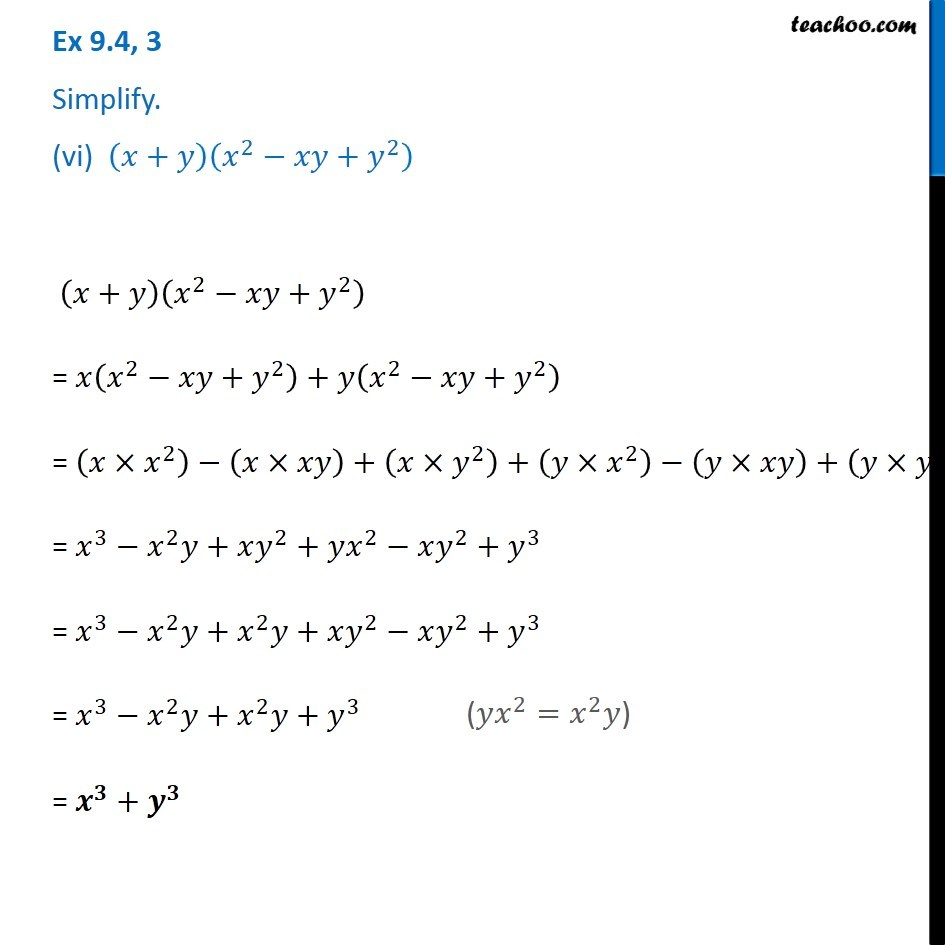Subscribe to our Youtube Channel - https://you.tube/teachoo

1. Chapter 9 Class 8 Algebraic Expressions and Identities
2. Concept wise
3. Multiplication of Polynoimals by Polynomials

Transcript

Ex 9.4, 3 Simplify. (vi) (𝑥+𝑦)(𝑥^2−𝑥𝑦+𝑦^2 ) (𝑥+𝑦)(𝑥^2−𝑥𝑦+𝑦^2 ) = 𝑥(𝑥^2−𝑥𝑦+𝑦^2 )+𝑦(𝑥^2−𝑥𝑦+𝑦^2 ) = (𝑥×𝑥^2 )−(𝑥×𝑥𝑦)+(𝑥×𝑦^2 )+(𝑦×𝑥^2 )−(𝑦×𝑥𝑦)+(𝑦×𝑦^2 ) = 𝑥^3−𝑥^2 𝑦+𝑥𝑦^2+𝑦𝑥^2−𝑥𝑦^2+𝑦^3 = 𝑥^3−𝑥^2 𝑦+𝑥^2 𝑦+𝑥𝑦^2−𝑥𝑦^2+𝑦^3 = 𝑥^3−𝑥^2 𝑦+𝑥^2 𝑦+𝑦^3 = 𝒙^𝟑+𝒚^𝟑 (𝑦𝑥^2=𝑥^2 𝑦)

Multiplication of Polynoimals by Polynomials